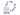# Logic is Like Abstract Art

Modern logic unleashed new areas of mathematics. Even when language and intuition fail, conclusive theories can be constructed with the help of logic.1/3 images [Translate to English:] Der Vienna Summer of Logic [Translate to English:] Der Vienna Summer of Logic [Translate to English:] Der Vienna Summer of Logic

When little children share chocolates, they already have to deal with numbers. Even complicated mathematical objects can often be grasped intuitively. We draw vectors as little arrows of a sheet of paper. But how can we draw a non-commutative algebra? How can we understand quaternions and tensor products, if we cannot make them out of chocolate? When one tries to deal with completely abstract mathematical objects without getting caught in contradictions, or when one wants to analyse mathematics itself with mathematical methods, logic is indispensable.

A Language for Talking about Math
“The question, what exactly constitutes a proof, is not really part of mathematics”, says Professor Matthias Baaz (Vienna University of Technology). In mathematics, people talk about particularly “beautiful proofs” or “trivially evident statements”. But mathematics does not supply any information on whether there could be an even more elegant line of reasoning to proof a statement. Logic goes one step further. “It saves mathematics from the mistakes which might arise due to flawed linguistic concepts”, says Matthias Baaz.

Logic has not always been that closely connected to mathematics. In the middle ages, it was considered a part of philosophy, a set of rules for proper verbal reasoning. But this changed dramatically in the twentieth century.

Representational and Abstract Mathematics
The mathematician David Hilbert was one of the most prominent advocates of logical methods in mathematics. He called for mathematics to be logically founded on a small set of simple basic assumptions. From these axioms, all of mathematics should logically follow, without leaving any room for verbal imprecisions. Even seemingly trivial statements should be formally proven, without referring to intuition. In their book “Principia Mathematica”, Bertrand Russell and Alfred Whitehead even proved the equation “1+1=2” with logical methods.

Hilbert had a dualistic view of mathematics: There is “realistic” mathematics, which can be intuitively grasped and is known a priory, in Immanuel Kant’s terms. Apart from that, there is also an abstract mathematics, a world of objects, which can be used employing the tools of logic, even if we do not have any intuitive understanding of them.

“This new kind of abstract mathematics emerged at the same time as abstract art”, says Matthias Baaz. “When the step towards abstraction is made, the basic techniques remain the same, but the relationship between the work and the world around us is changed.”

Intersecting Parallels
The relationship between representational and abstract mathematics can be studied on the basis of the famous parallel postulate: the Greek mathematician Euclid of Alexandria developed a geometry based on simple axioms – in much the same way as Hilbert wanted to structure all of mathematics many years later. Euclid’s fifth axiom, howewer, did not sound quite as evident as the others. In modern terms, it says: “For any given line, there is exactly one parallel line through one given point.”

Is this axiom really necessary for geometry? Addressing this question in terms of representational mathematics, this leads to completely new kinds of geometry – such as spherical geometry, in which all great circles intersect at some point and all triangles have an angular sum of more than 180 degrees. In this case, the notion that Euclid’s fifth axiom is not really necessary comes from the construction of a new geometric reality, which in itself can again be grasped intuitively.

But actually, constructing such alternative geometries is not really necessary. Using the tools of logic, one can check whether certain assumptions are compatible, whether they might contradict each other at some point, or whether one of them is perhaps not even an additional assumption, because it follows logically from the others. “From a logic point of view, we can just say: Euclid’s fifth axiom cannot be derived from the others, and both the fifth axiom and its opposite are compatible with the other axioms”, says Matthias Baaz. “This means that in a way, you can decide for yourself whether you hold the parallel postulate to be true or not. Intuitively speaking, from this choice different kinds of geometry follow, the Euclidean geometry of flat planes or different ones.”

Whole theories can be checked for consistency. In mathematics, this is very important – if there was a true statement with an opposite that can also be shown to be true, the theory would break down. But consistency is also crucial for other areas, for instance in legal systems. For studying theories and their consistency, modern logic provides many different tools. In addition to classical logic, different logical systems are used today, for instance logics that do not only operate with the values “true” or “false”, but can also deal with shades in between, or modal logic, which can express modalities such as “necessarily” or “possibly”: a circle is necessarily round, whereas a table is not necessarily rectangular.

A Brave New World
“Logic has unleashed mathematics”, says Matthias Baaz. Still, he has the impression, that some mathematicians are reluctant to embrace logic. But once one has taken on the adventure of logic, one does not only discover new mathematical statements that describe our world, but one is swept into new mathematical worlds, in which contradictions and inconsistencies can be safely contained. And who would not want that!

Read more about the Vienna Summer of Logic on the conference webpage: http://vsl2014.at/

Further Information:
Prof. Matthias Baaz
Institute of Discrete Mathematics and Geometry
Vienna University of Technology
Wiedner Hauptstraße 8-10, 1040 Vienna
T: +43-1-58801-10420
matthias.baaz@tuwien.ac.at